### Site Information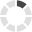Loading... Please wait...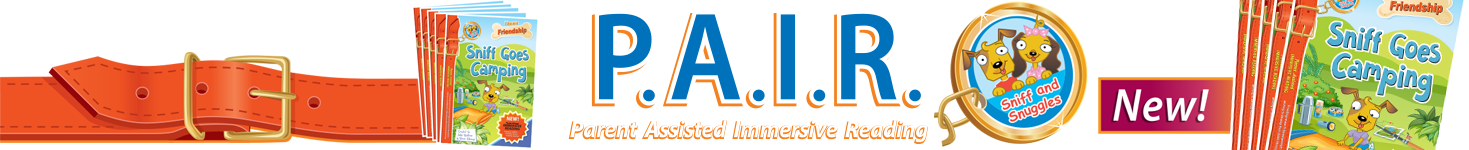# Problem Solved

## Product Description

### Engaging multi-levelled problem-solving series to support the Australian Curriculum

Problem Solved is an innovative mathematical problem-solving series providing students with opportunities to apply their numeracy skills in order to solve problems. The problem-solving process will vary from student to student and from problem to problem.

## Key Features

As students analyse the problems in Problem Solved they will be required to determine:

• what the problem is asking
• what strategy best suits the problem
• what mathematical knowledge needs to be employed
• whether the solution makes sense

Throughout the series, students are encouraged to use a variety of different problem-solving strategies, such as:

• estimating
• discussions
• using calculators
• using materials
• creating algorithms
• working backwards
• creating tables and lists

Content areas include:

• whole number
• operations
• fractions and decimals
• patterns and algebra
• measurement
• geometry
• statistics and probability

The problems presented on each page are arranged in three levels.

\$12.95 (inc. GST)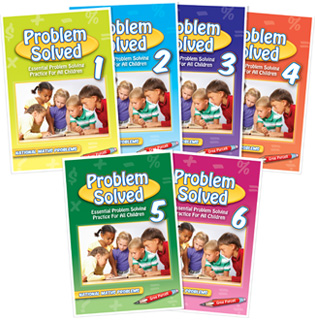Problem Solved 1

Problem Solved 1

Problem Solved 2

Problem Solved 2

Problem Solved 3

Problem Solved 3

Problem Solved 4

Problem Solved 4

Problem Solved 5

Problem Solved 5

Problem Solved 6

Problem Solved 6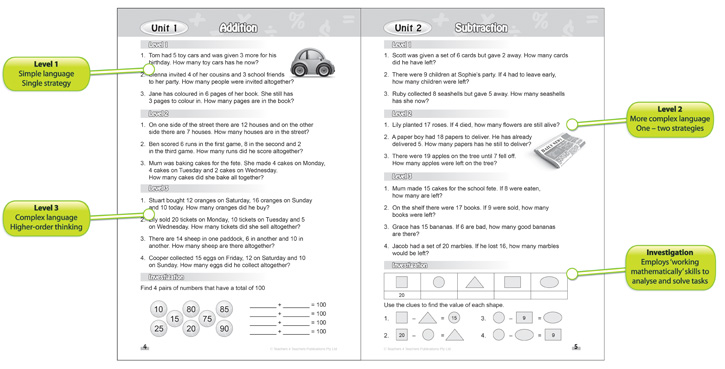## Related Products

• Enhancement Maths \$19.95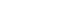• Maths Box Aqua \$897.60• Maths Box Orange \$897.60• Maths Box Lime \$897.60